# PlotPub-v1.4 Documentation

## Tutorial

To create a beautiful figure using PlotPub, all you need to know is how to use the set-plot-properties (setPlotProp) function. It has the following syntax:

function h = setPlotProp(opt, hfig)


where, opt is the options structure that controls the figure properties and hfig is figure handle.

Let us walk you through an example. Assume that we have data for 3 cycle of a 50 Hz AC voltage signal:

clear all;

%% lets plot 3 cycles of 50Hz AC voltage
f = 50; % frequency
Vm = 10; % peak
phi = 0; % phase

% generate the signal
t = [0:0.0001:3/f];
th = 2*pi*f*t;
v = Vm*sin(th+phi);

% plot it
figure;
plot(t*1E3, v);


where, f is the frequency, Vm is the peak voltage, t is time and v is the AC voltage signal. Result? An utterly ugly looking figure punching at your face: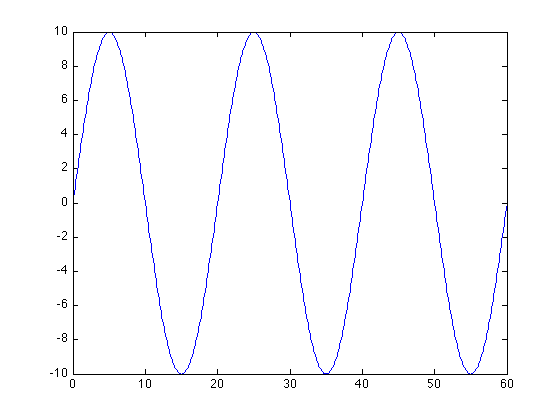Now, let us add some spices. Let us set the labels:

% change settings
opt = [];
opt.XLabel = 'Time, t (ms)'; % xlabel
opt.YLabel = 'Voltage, V (V)'; % ylabel


If we want to save the plot in a file, we have to assign a file name:

% Save? comment the following line if you do not want to save
opt.FileName = 'plotSimple1.png';


Finally, call the setPlotProp function to apply the settings and export it:

% apply the settings
setPlotProp(opt);


The resulting plot should look like: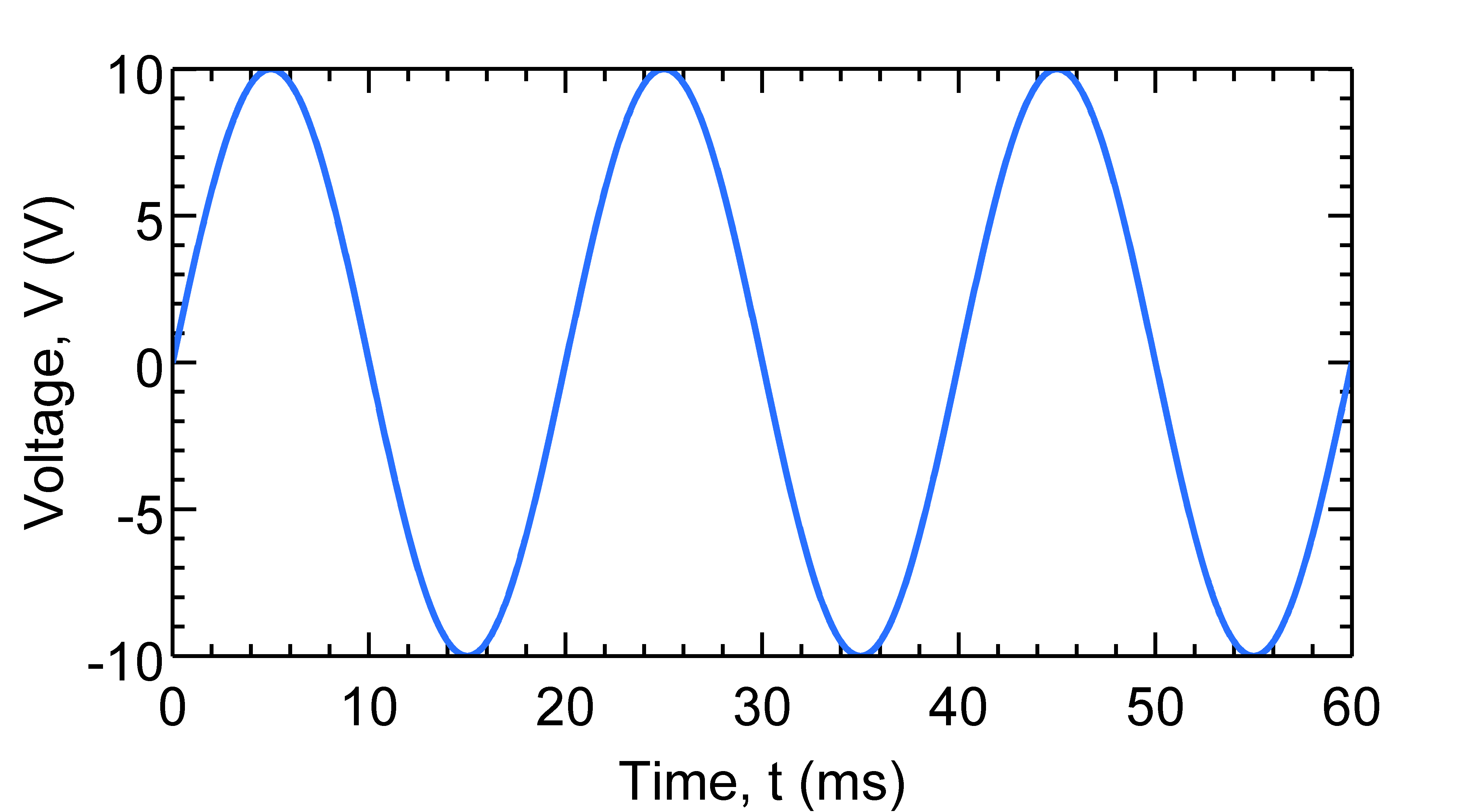The full source code for this plot, plotSimple.m, can be found inside the examples folder.

We can change color, linewidth, linestyle etc:

opt.Colors = [0, 0, 0]; % [red, green, blue]
opt.LineWidth = 2; % line width
opt.LineStyle = {'--'}; % line style: '-', ':', '--' etc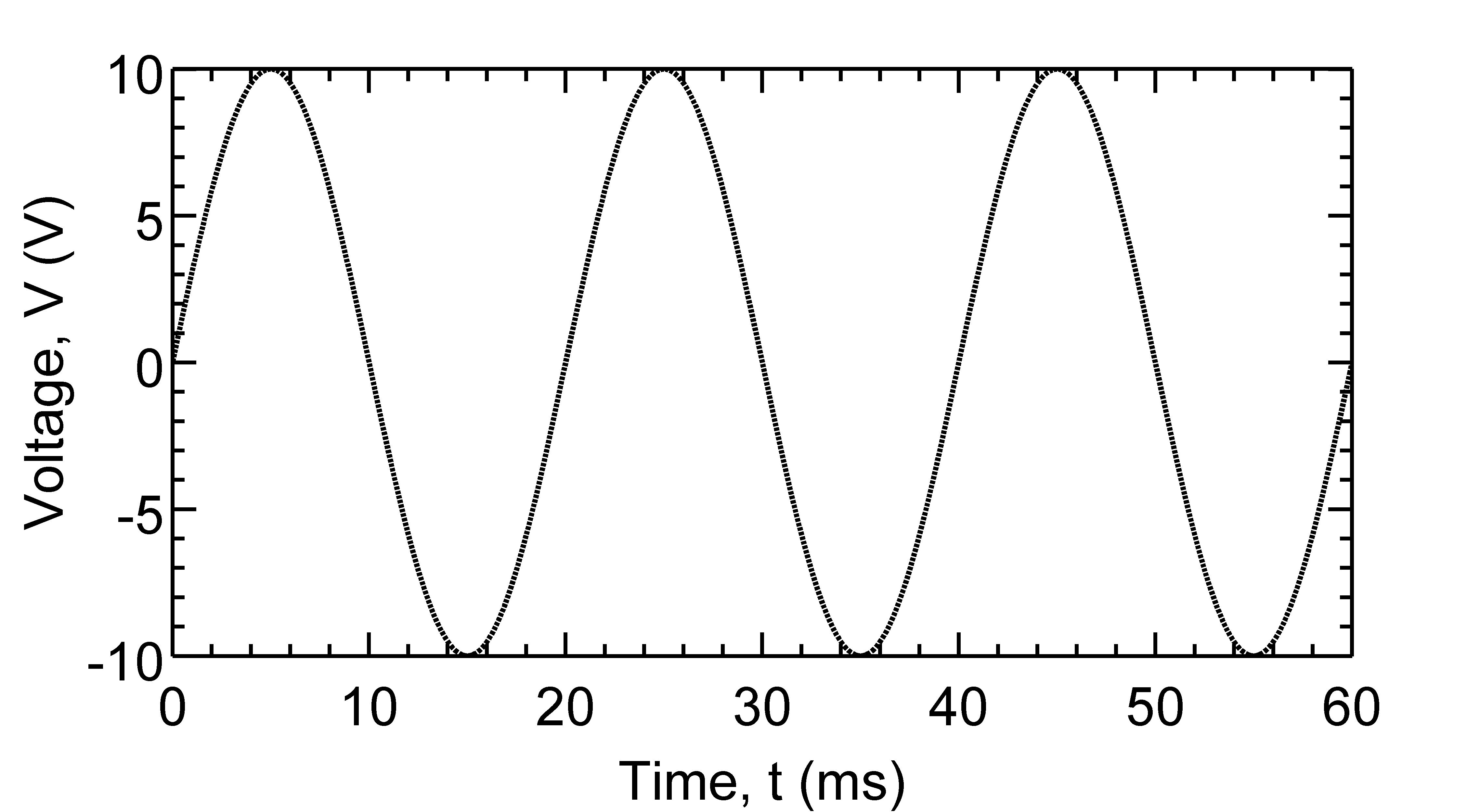See plotLineStyle.m for full source code. We can also change scale, axis limit, tick and grid:

opt.YScale = 'log'; % 'linear' or 'log'
opt.YLim = [1E-3, 1E3]; % [min, max]
opt.YTick = [1E-3, 1E-1, 1E1, 1E3]; %[tick1, tick2, .. ]
opt.YGrid = 'on'; % 'on' or 'off'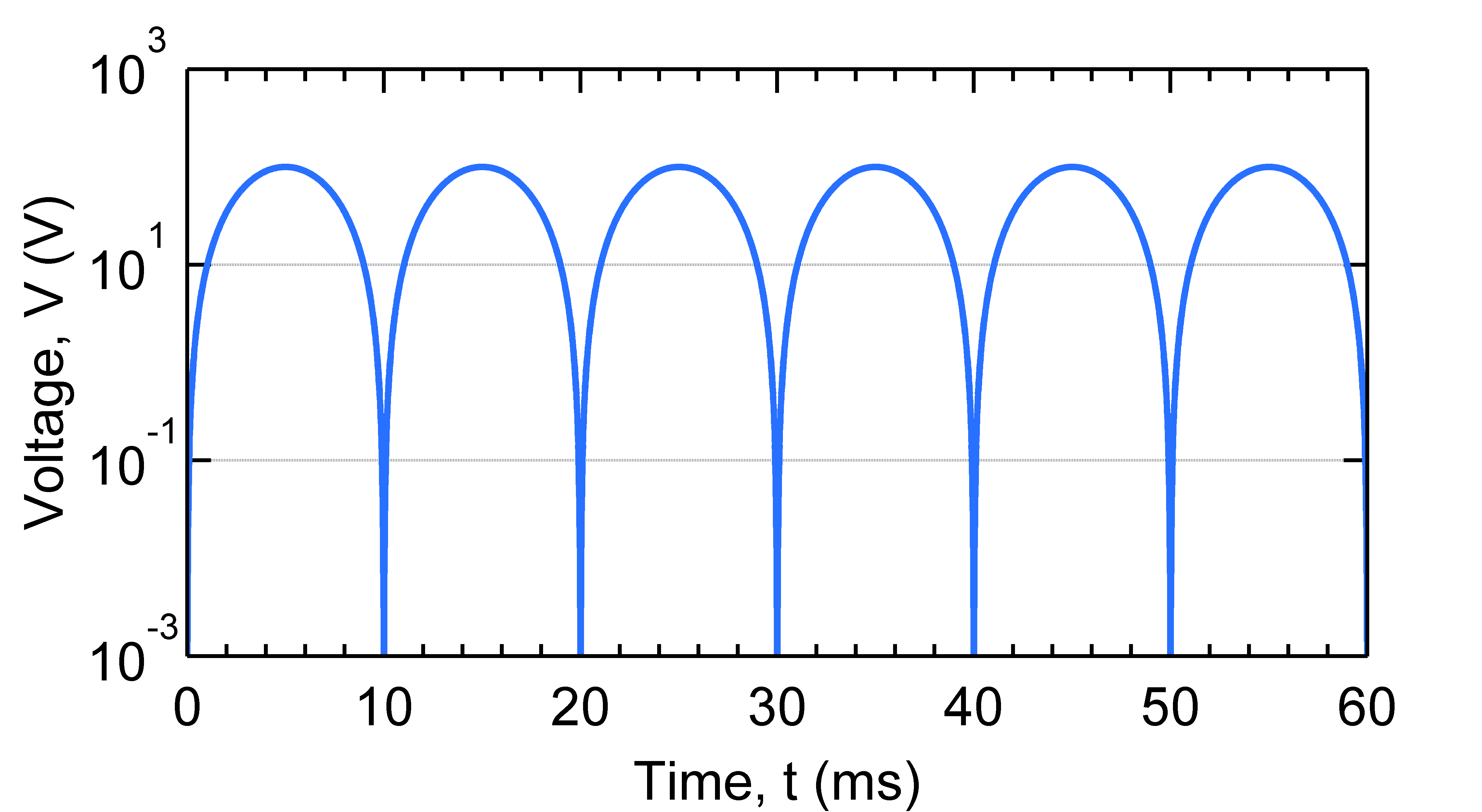or create a log-log plot:

opt.YScale = 'log'; % 'linear' or 'log'
opt.XScale = 'log'; % 'linear' or 'log'
opt.YLim = [1E-3, 1E3]; % [min, max]
opt.YTick = [1E-3, 1E-1, 1E1, 1E3]; %[tick1, tick2, .. ]
opt.YGrid = 'on'; % 'on' or 'off'
opt.XGrid = 'on'; % 'on' or 'off'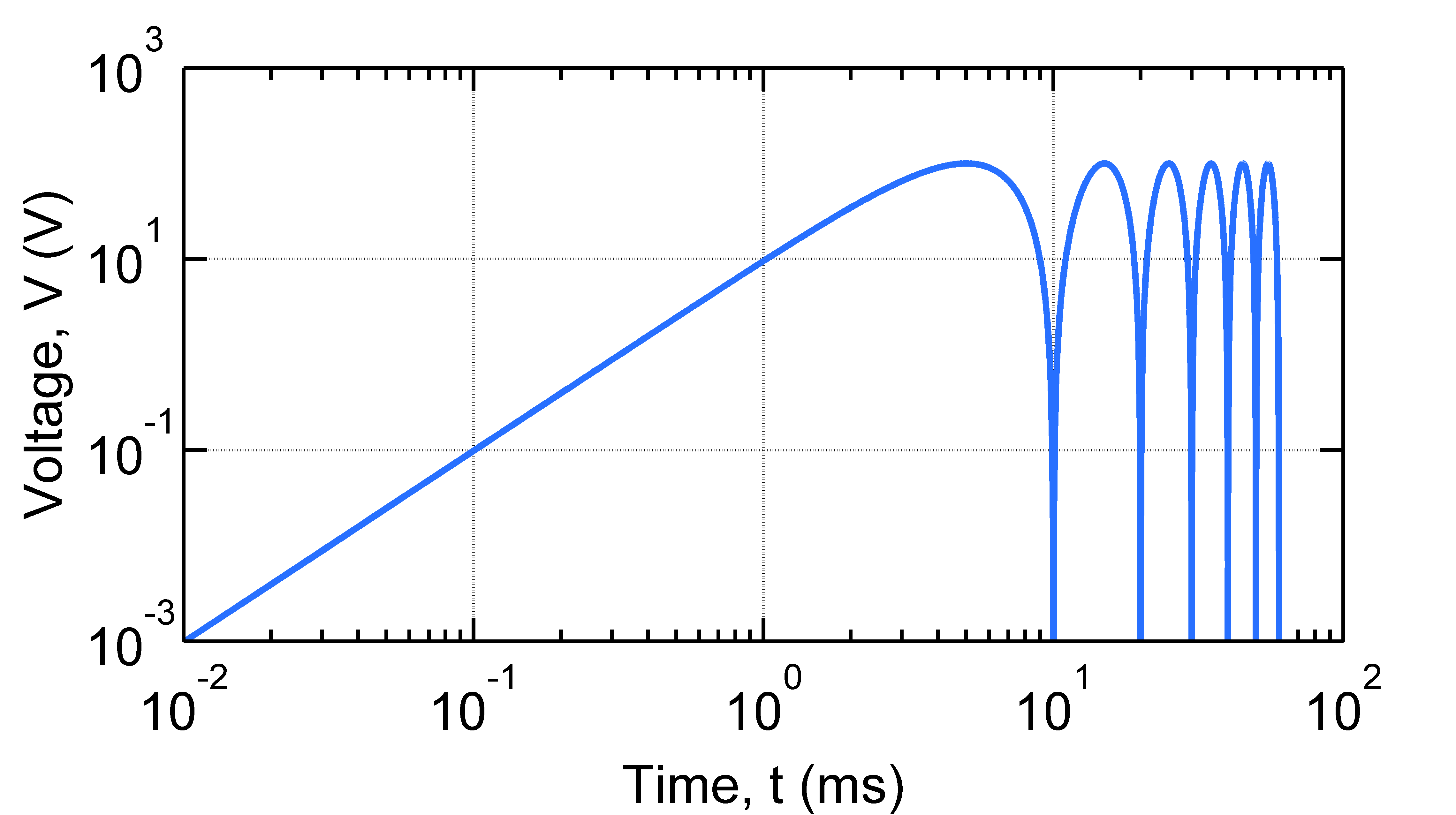See plotSimpleLog.m and plotSimpleLogLog.m for full source code.

Creating multiple plots in a single set of axes is also easy:

% generate the signal
t = [0:0.0001:3/f];
th = 2*pi*f*t;
v1 = Vm*sin(th);
v2 = Vm*sin(th - phi);
v3 = Vm*sin(th - phi*2);

% plot them
figure;
plot(t*1E3, v1);
hold on;
plot(t*1E3, v2);
plot(t*1E3, v3);
hold off;

% change settings
opt = [];
opt.XLabel = 'Time, t (ms)'; % xlabel
opt.YLabel = 'Voltage, V (V)'; % ylabel
opt.YTick = [-10, 0, 10]; % [tick1, tick2, .. ]
opt.YLim = [-11, 11]; % [min, max]

% Save? comment the following line if you do not want to save
opt.FileName = 'plotMultiple.png';

% apply the settings
setPlotProp(opt);


result: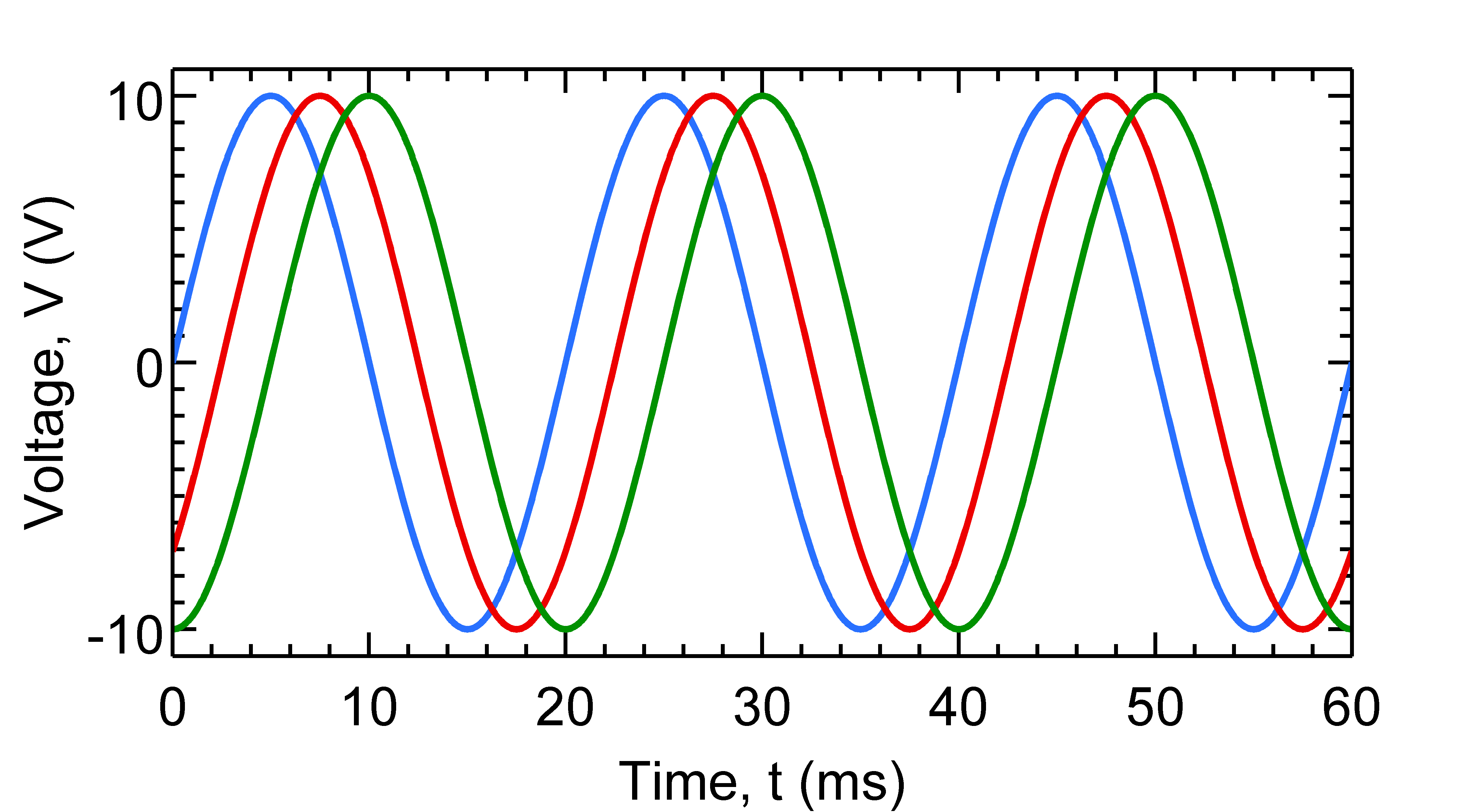The full source is given in plotMultiple.m. We can change the linestyle, color etc and add a legend:

opt.XLim = [0, 80]; % [min, max]
opt.Colors = [ % three colors for three data set
1, 0, 0; % data set 1
0.25, 0.25, 0.25; % data set 2
0, 0, 1; % data set 3
];
opt.LineWidth = [2, 2, 2]; % three line widths
opt.LineStyle = {'-', '-', '-'}; % three line styles
opt.Markers = {'o', '', 's'};
opt.MarkerSpacing = [15, 15, 15];
opt.Legend = {'\theta = 0^o', '\theta = 45^o', '\theta = 90^o'}; % legends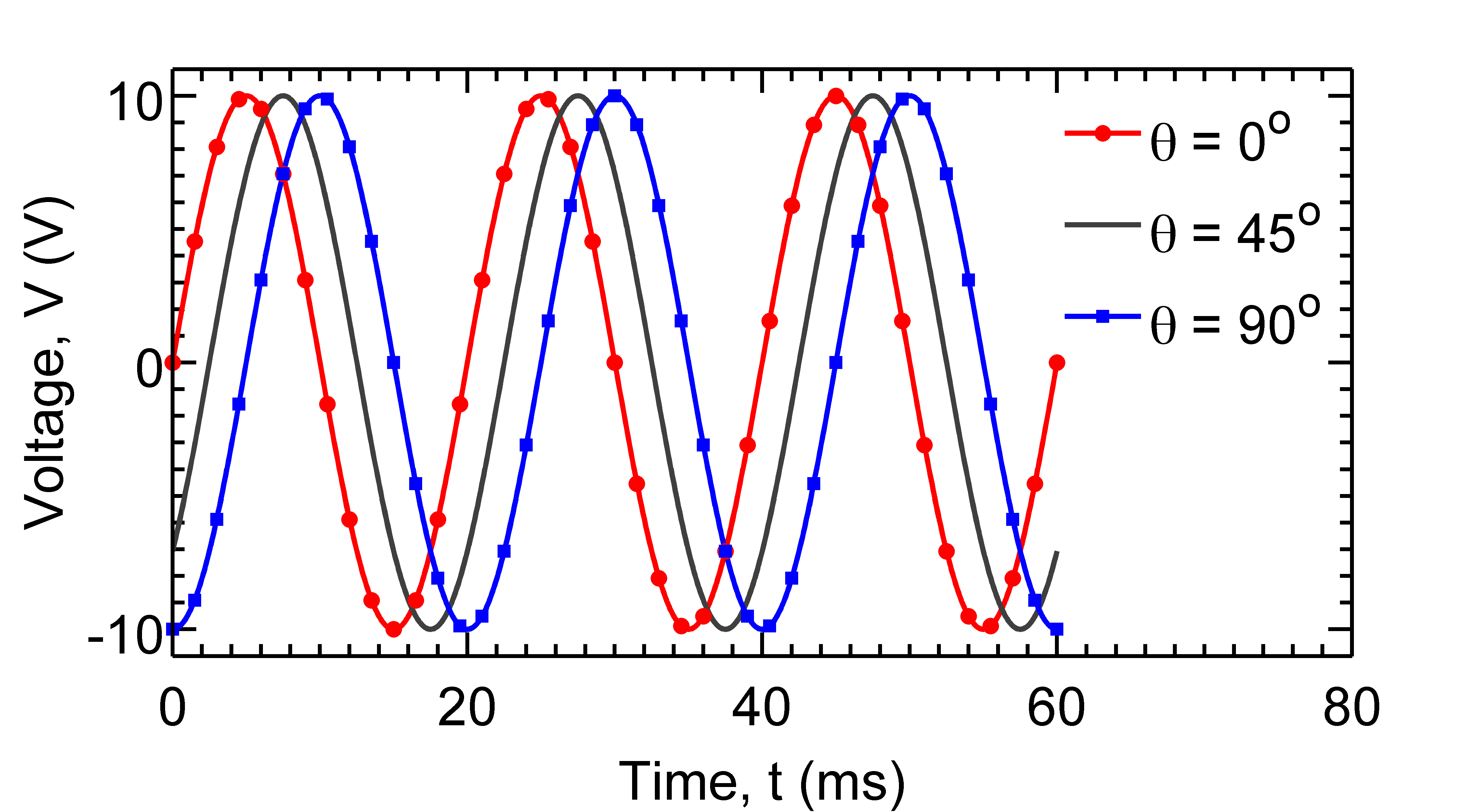Here, opt.Colors(1,:), opt.LineWidth(1) and opt.LineStyle{1} set properties of data set 1 and so on. The full source is given in plotMarkers.m.

By default, plotPub creates figures with 6in x 2.5in box size. You can easily change the figure size using the following code.

opt.BoxDim = [7, 3]; %[width, height] in inches


This code creates a figure with 7in x 3in box.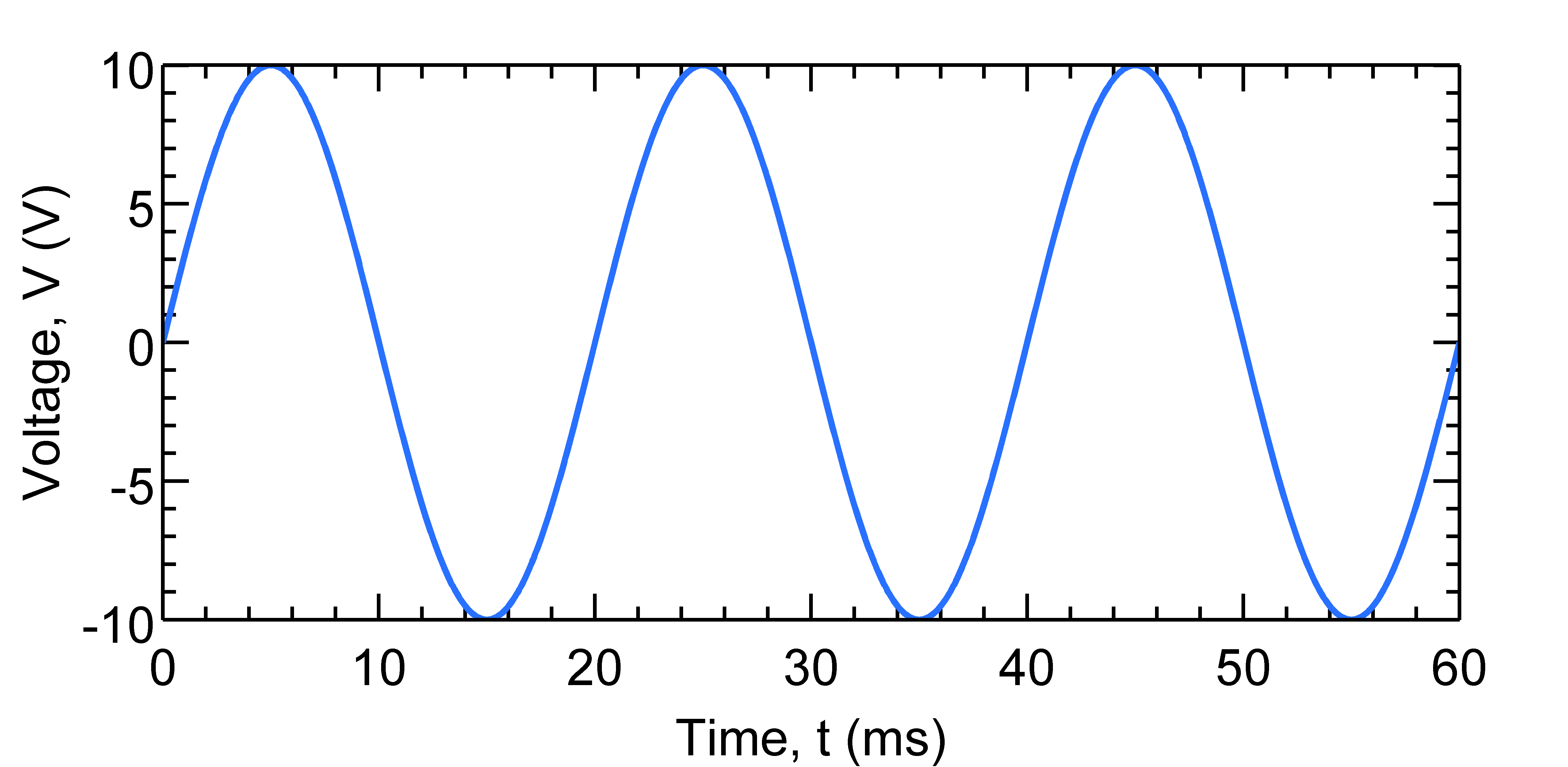See plotSize.m for more details.

You can also load a previously saved MATLAB fig file and export it using setPlotProp:

clear all;

% load previously generated fig file
figFile = 'single.fig';
open(figFile)

% change settings
opt.XLabel = 'Time, t (ms)'; % xlabel
opt.YLabel = 'Voltage, V (V)'; %ylabel
opt.BoxDim = [6, 5]; %[width, height]

% Save? comment the following line if you do not want to save
opt.FileName = 'plotSize.png';

% apply
setPlotProp(opt);


## Documentation

Given bellow is a brief description of setPlotProp and plotPub functions and their parameters. This documents can also be viewed by invoking:

>> help setPlotProp


from inside the MATLAB command window.

### function h = setPlotProp(opt, hfig)

This function changes the properties of the figure represented by 'hfig' and exports it as a publication quality image file. The resolution of the image can be chosen by the user. Supported image formats are EPS, PDF, PNG, JPEG and TIFF. The figure properties are specified by the options structure 'opt'.

Parameters:

opt % options structure:
BoxDim % vector [width, height]: size of the axes box in inches; default: [6, 2.5]
ShowBox % 'on' = show or 'off' = hide bounding box; default: 'on'
FontName % string: font name; default: 'Arial'
FontSize % integer; default: 26
LineWidth % vector [width1, width2, ..]: element i changes the property of i-th dataset; default: 2
LineStyle % cell array {'style1', 'style2', ..}: element i changes the property of i-th dataset; default: '-'
Markers % cell array {'marker1', 'marker2', ..}: element i changes the property of i-th dataset; default: 'None'
MarkerSpacing % vector [space1, space2, ..]: element i changes the property of i-th dataset; default: 0
Colors % 3xN matrix, [red, green, blue] where N is the number of datasets.
AxisColor % [red, green, blue]; color of the axis lines; default: black
AxisLineWidth % Witdth of the axis lines; default: 2
XLabel % X axis label
YLabel % Y axis label
ZLabel % Z axis label
XTick % [tick1, tick2, ..]: major ticks for X axis.
YTick % [tick1, tick2, ..]: major ticks for Y axis.
ZTick % [tick1, tick2, ..]: major ticks for Z axis.
XMinorTick % 'on' or 'off': show X minor tick?
YMinorTick % 'on' or 'off': show Y minor tick?
ZMinorTick % 'on' or 'off': show Z minor tick?
TickDir % tick direction: 'in' or 'out'; default: 'in'
TickLength % tick length; default: [0.02, 0.02]
XLim % [min, max]: X axis limit.
YLim % [min, max]: Y axis limit.
ZLim % [min, max]: Z axis limit.
XScale % 'linear' or 'log': X axis scale.
YScale % 'linear' or 'log': Y axis scale.
ZScale % 'linear' or 'log': Z axis scale.
XGrid % 'on' or 'off': show grid in X axis?
YGrid % 'on' or 'off': show grid in Y axis?
ZGrid % 'on' or 'off': show grid in Z axis?
XDir % 'in' or 'out': X axis tick direction
YDir % 'in' or 'out': Y axis tick direction
ZDir % 'in' or 'out': Z axis tick direction
Legend % {'legend1','legend2',...}
LegendBox % bounding box of legend: 'on'/'off'; default: 'off'
LegendBoxColor % color of the bounding box of legend; default: 'none'
LegendTextColor % color of the legend text; default: [0,0,0]
LegendLoc % 'NorthEast', ..., 'SouthWest': legend location
Resolution % Resolution (dpi) for bitmapped file. Default:600.
HoldLines % true/false. true == only modify axes settings, do not touch plot lines/surfaces. Default false.
FileName % Save? Give a file name.

hfig % Figure handle (optional). Default: current figure.


Return value: figure handle.

### function h = plotPub(X, Y, N, opt)

This function plots X{i} vs Y{i}, changes the properties of the generated figure and exports it as a publication quality image file. The resolution of the image can be chosen by the user. Supported image formats are EPS, PDF, PNG, JPEG and TIFF. The figure properties are specified by the options structure 'opt'.

Parameters:

X %cell array of x coordinates
Y %cell array of y coordinates
N %number of plots to be created. N &amp;lt;= length(X)
opt %options structure. Same as above.


Last update: 2:24 PM, Mar 19, 2015.